## 华丽丽的换代 全新宝马3系官图解析

2014年04月10日 16:06 来源：www.chextx.com 超过：次关注

将宝马3系称为运动轿车的标杆一点都不为过，这不仅仅是一个口号，也是积累的口碑，完美的前后质量配比、精准的转向、出色底盘调教功底，宝马3系从设计初衷到实际表现都是围绕着“运动”二字，在同级车里的性能表现也堪称优秀，它每一次升级换代都会引起很大的轰动，全新一代3系车型在前几日在海外发布，全球无数的媒体和车迷都将目光都投向了它的身上，尽管新车上市还要过段时间，但我们已经可以通过清晰的官方图片来详细的打量一番。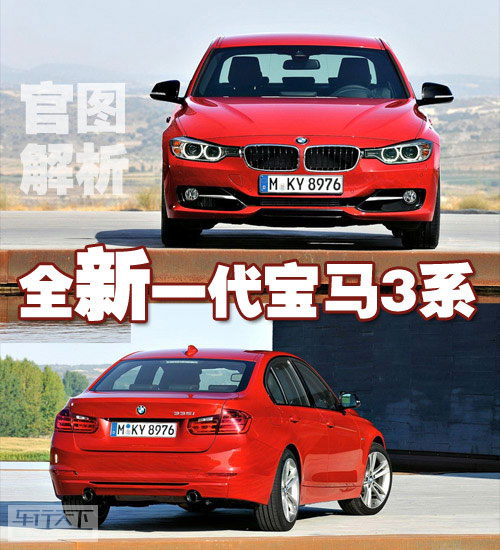第一代宝马3系（E21）诞生于1975年，此次新发布的为第6代车型，它将会有哪些吸引人的亮点呢？让我们先从外形说起。宝马3系每一次换代都会带来不少喝彩、议论甚至是争议，就像第6代车型的外观一样，人们对它的看法褒贬不一，尤其是与中网连在一起的大灯是大家讨论最多的话题。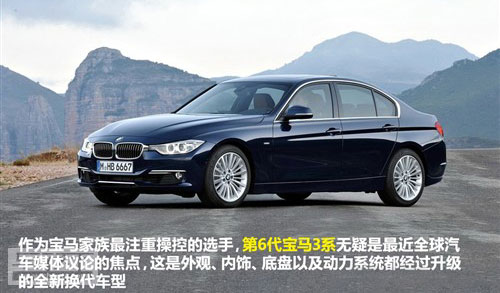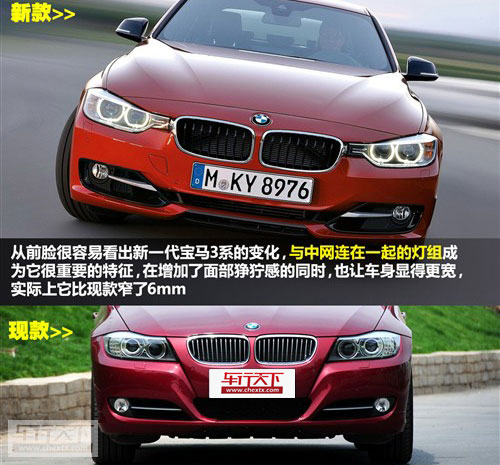无论您觉得好看还是不好看，它就是这么设计出来，如果您喜欢3系就得接受这样的前脸，新式样的灯组让前脸显得更宽，双肾的进气格栅变得更大，因此面部的狰狞感也更为强烈。如果您觉得新设计不满意，那么也完全没有必要去指着它，每次全新的造型都会引起部分人的排斥，事实证明对宝马而言，通常不存在好不好看，而是习不习惯的问题，当年的7系也是遭到不少非议，而如今身上的很多元素却成为其他厂商效仿的对象。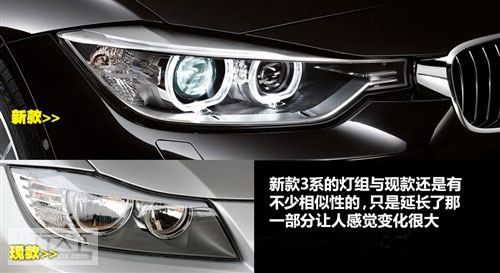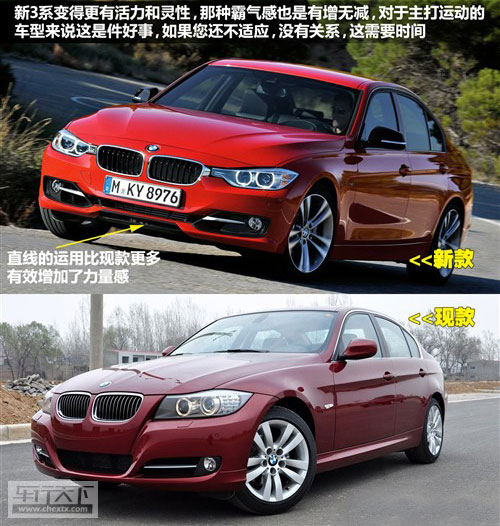新3系灯组的样式还是继承了现款车型大致轮廓的，只是向中网延长了一部分让人感觉变化很大，前脸也运用了更多的直线，以此来增强力量感。第一次看到新3系的时候，我对前脸设计也略有不适应，但与老款照片一对比很容易发现，新车型的明显更加具有活力，在气势上也更容易让人联想到生猛和运动之类的词汇。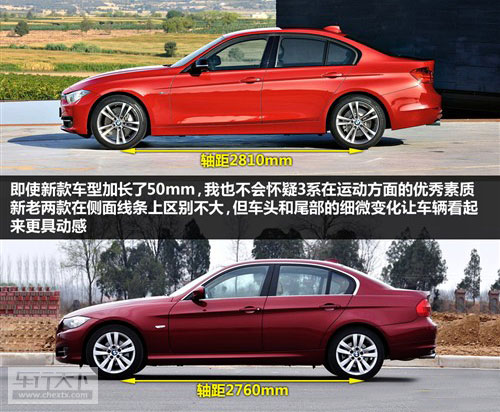将新款与现款车型在侧面车身线条上基本一致，但是新3系略微下沉的车头造型和尾部的小变化让人觉得更有动感。新款车型除了宽度比现款略窄外，长度和宽度方面都有提升，轴距也增加了50mm而达到2810mm，加长后的操控能力是否有所下降还有待考证，不过厂商也强调新3系的后排空间加长了15mm，头部空间增加了8mm，看来宝马此次也更加关心乘坐需求。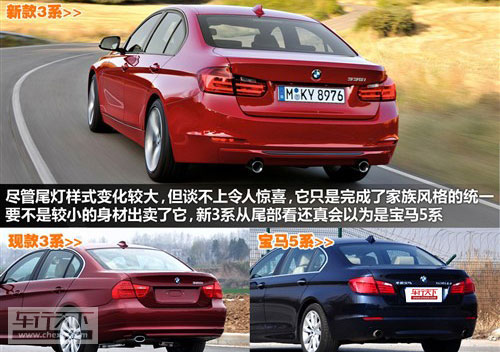全新宝马3系的尾部设计也达到了家族化的统一，尤其是尾灯造型大家并不陌生，远看起来与5系车型非常相似，如果不是因为3系的车身会显得小巧一些，还真会以为是5系来了，相比现款3系尾灯的设计，新款车型削减了些锐气而变得更为阴柔，融入整车的线条也很合拍。总的来讲，全新的宝马3系的外形改变还是很有看头的，年轻和活力将继续围绕着新一代3系车型。

看完了外观，我们再来看看内饰。相信你那对宝马家族式的风格也早已熟悉，新3系也没能有什么突破，它融入了一些5系的元素进去，坦白的讲，新3系的豪华气息有所加强，让档次感进一步提高。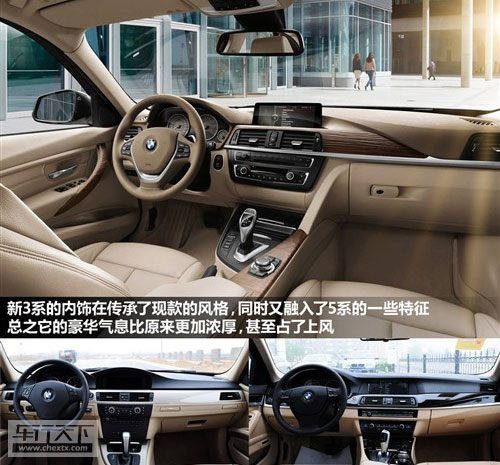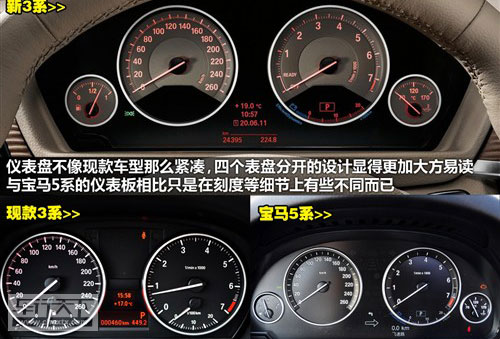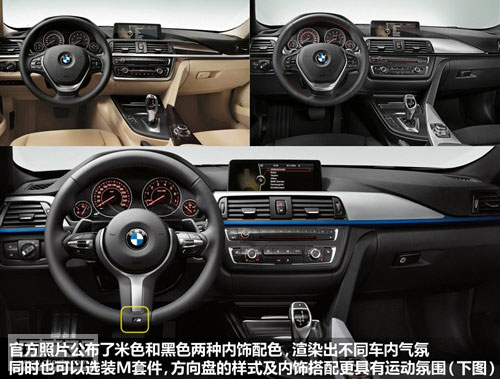尽管3系轿车的运动基因不会改变，但内饰太运动化也未必是件好事，毕竟不是所有购买3系的热都是狂热的运动分子，这种偏重豪华的取向其实挺好的。上面已经提到有些地方很像5系，比如仪表设计就是如此，除了细节方面，新3系的仪表几乎与5系的完全相同，相比现款显得大方许多，同时也更为易读，但似乎少了一些宝马的经典机械美感。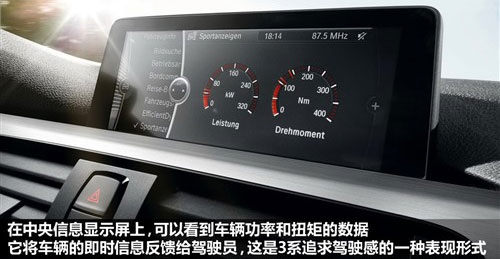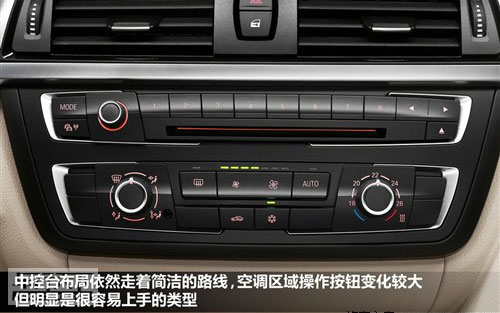在中央信息显示屏幕上，功率和扭矩的显示已经作为i-Drive的一项功能，它可以让车主了解车辆当前的即时动力输出，我认为这也是追求运动的一种表现形式。新3系中控台的布局仍然非常简洁，空调面板相比现款车型有点变化，但功能上还是一目了然。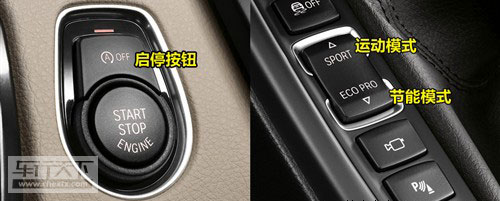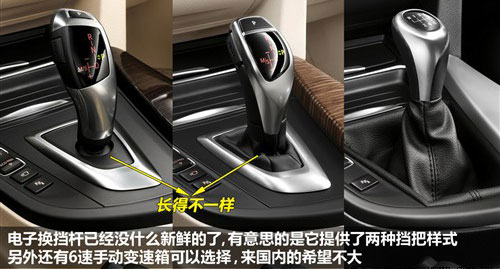新3系将配备的启停系统、车辆驾驶模式选择和电子换挡杆在宝马上也不是第一次出现了，有意思的是，从官图上看新3系提供了两种自动挡挡把的样式。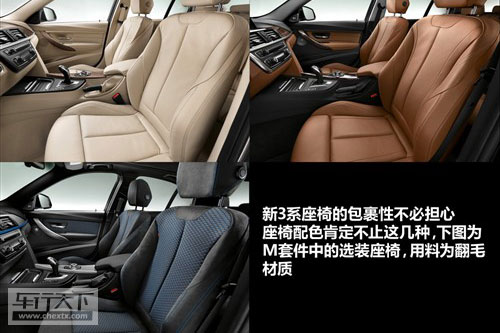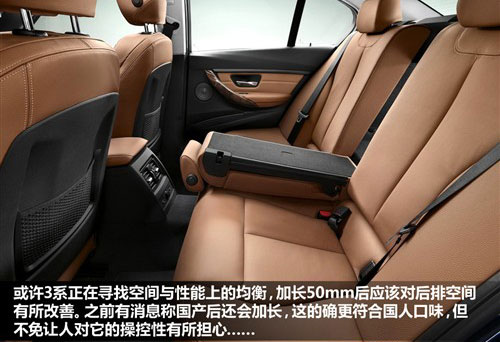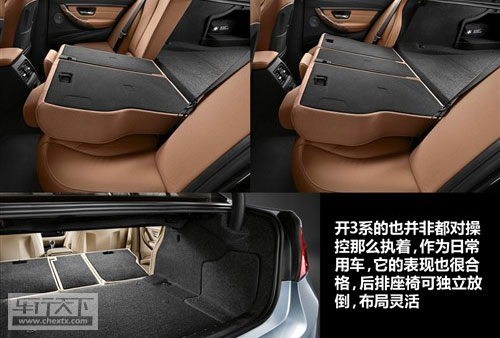我对新3系的座椅包裹能力丝毫不会怀疑，因为这是它的专长，可以选装的M套件里包含翻毛材质的座椅，可以提供更大的摩擦力。加长50mm后，后排空间的局促感会有所改善，同时后排座椅可以分别独立放倒，在实用性方面做得也非常到位。可以说，新款3系的四门版或许是试图在寻找运动与乘坐之间的最佳平衡点。新款3系的底盘结构与现款相同，前后比重仍然是完美的50:50，汽油动力方面，它全系都采用涡轮增压发动机，与之匹配的8速手自一体或6速手动变速箱，当然了，手动变速箱来国内的机会很小，不过回顾下以前的车型，这倒也不是不可能。据悉，宝马在后期还会推出四驱版和混合动力版本的3系，在提升自身性能的同时也会增加产品的竞争力。

#### 相关文章

﻿
• 快速找车
• 选择品牌
• 选择品牌
• A  奥迪
• A  阿斯顿·马丁
• A  阿尔法·罗密欧
• B  宝沃
• B  布加迪
• B  巴博斯
• B  保时捷
• B  宾利
• B  奔驰
• B  宝马
• B  本田
• B  别克
• B  标致
• B  比亚迪
• B  宝骏
• B  北汽制造
• B  北汽新能源
• B  北汽幻速
• B  北汽威旺
• B  北京汽车
• B  奔腾
• B  北汽绅宝
• B  北汽昌河
• C  长安欧尚
• C  长安
• C  长安凯程
• C  长城
• D  大众
• D  道奇
• D  DS
• D  东南
• D  东风风神
• D  东风风行
• D  东风小康
• D  东风风度
• D  东风
• F  福特
• F  丰田
• F  菲亚特
• F  法拉利
• F  福田
• F  福迪
• F  福汽启腾
• G  观致
• G  广汽传祺
• G  广汽吉奥
• G  GMC
• H  红旗
• H  汉腾汽车
• H  哈弗
• H  哈飞
• H  海格
• H  海马
• H  华颂
• H  黄海
• H  华泰
• H  恒天
• J  捷途
• J  几何汽车
• J  捷达
• J  吉利汽车
• J  捷豹
• J  Jeep
• J  江淮
• J  江铃
• J  金杯
• J  九龙
• J  金旅
• K  凯翼
• K  凯迪拉克
• K  克莱斯勒
• K  科尼塞克
• K  卡威
• K  开瑞
• L  路虎
• L  林肯
• L  劳斯莱斯
• L  兰博基尼
• L  雷克萨斯
• L  铃木
• L  领克
• L  雷诺
• L  理念
• L  力帆
• L  莲花汽车
• L  猎豹
• L  路特斯
• L  陆风
• M  马自达
• M  MG
• M  MINI
• M  玛莎拉蒂
• M  摩根
• M  迈凯轮
• N  纳智捷
• O  欧拉
• O  欧宝
• O  讴歌
• O  欧朗
• Q  奇瑞
• Q  起亚
• Q  启辰
• R  日产
• R  荣威
• R  瑞麒
• S  SERES赛力斯
• S  三菱
• S  斯威汽车
• S  萨博
• S  smart
• S  斯柯达
• S  斯巴鲁
• S  思铭
• S  双龙
• S  上汽大通
• S  双环
• T  特斯拉
• T  腾势
• W  蔚来
• W  沃尔沃
• W  WEY
• W  五菱汽车
• W  五十铃
• W  威兹曼
• W  威麟
• X  现代
• X  雪佛兰
• X  星途
• X  雪铁龙
• X  小鹏汽车
• X  西雅特
• Y  一汽
• Y  英菲尼迪
• Y  英致
• Y  依维柯
• Y  野马汽车
• Y  永源
• Z  众泰
• Z  中华
• Z  中兴
• Z  知豆
• 选择车系
• 选择车系
• 车型对比
• 选择品牌
• 选择品牌
• A  奥迪
• A  阿斯顿·马丁
• A  阿尔法·罗密欧
• B  宝沃
• B  布加迪
• B  巴博斯
• B  保时捷
• B  宾利
• B  奔驰
• B  宝马
• B  本田
• B  别克
• B  标致
• B  比亚迪
• B  宝骏
• B  北汽制造
• B  北汽新能源
• B  北汽幻速
• B  北汽威旺
• B  北京汽车
• B  奔腾
• B  北汽绅宝
• B  北汽昌河
• C  长安欧尚
• C  长安
• C  长安凯程
• C  长城
• D  大众
• D  道奇
• D  DS
• D  东南
• D  东风风神
• D  东风风行
• D  东风小康
• D  东风风度
• D  东风
• F  福特
• F  丰田
• F  菲亚特
• F  法拉利
• F  福田
• F  福迪
• F  福汽启腾
• G  观致
• G  广汽传祺
• G  广汽吉奥
• G  GMC
• H  红旗
• H  汉腾汽车
• H  哈弗
• H  哈飞
• H  海格
• H  海马
• H  华颂
• H  黄海
• H  华泰
• H  恒天
• J  捷途
• J  几何汽车
• J  捷达
• J  吉利汽车
• J  捷豹
• J  Jeep
• J  江淮
• J  江铃
• J  金杯
• J  九龙
• J  金旅
• K  凯翼
• K  凯迪拉克
• K  克莱斯勒
• K  科尼塞克
• K  卡威
• K  开瑞
• L  路虎
• L  林肯
• L  劳斯莱斯
• L  兰博基尼
• L  雷克萨斯
• L  铃木
• L  领克
• L  雷诺
• L  理念
• L  力帆
• L  莲花汽车
• L  猎豹
• L  路特斯
• L  陆风
• M  马自达
• M  MG
• M  MINI
• M  玛莎拉蒂
• M  摩根
• M  迈凯轮
• N  纳智捷
• O  欧拉
• O  欧宝
• O  讴歌
• O  欧朗
• Q  奇瑞
• Q  起亚
• Q  启辰
• R  日产
• R  荣威
• R  瑞麒
• S  SERES赛力斯
• S  三菱
• S  斯威汽车
• S  萨博
• S  smart
• S  斯柯达
• S  斯巴鲁
• S  思铭
• S  双龙
• S  上汽大通
• S  双环
• T  特斯拉
• T  腾势
• W  蔚来
• W  沃尔沃
• W  WEY
• W  五菱汽车
• W  五十铃
• W  威兹曼
• W  威麟
• X  现代
• X  雪佛兰
• X  星途
• X  雪铁龙
• X  小鹏汽车
• X  西雅特
• Y  一汽
• Y  英菲尼迪
• Y  英致
• Y  依维柯
• Y  野马汽车
• Y  永源
• Z  众泰
• Z  中华
• Z  中兴
• Z  知豆
• 选择车系
• 选择车系
• 选择车型
• 选择车型
• 意见反馈